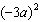Name:    Exponents Check Up

Multiple Choice
Identify the choice that best completes the statement or answers the question.

1.

Express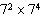as a single power.
 a.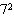b.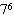c.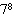d.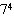2.

Express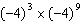as a single power.
 a.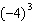b.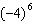c.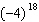d.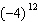3.

Evaluate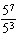.
 a. 5 b. 25 c. 125 d. 625

4.

Evaluate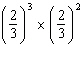.
 a.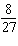b.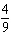c.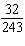d.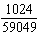5.

Evaluate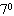.
 a. 0 b. 1 c. 7 d. 70

6.

Which is the simplified form of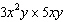?
 a.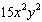c.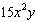b.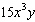d.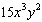7.

Which is the simplified form of g ´ g4 ´ g3?
 a. g7 c. g12 b. g8 d. g13

8.

What is the value of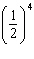?
 a.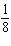c.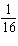b.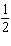d. 2

9.

The expressions in which pair are equal?
 a.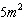and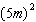c.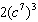and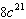b.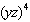and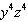d.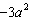and